# The MODEL Procedure

### Input Data Sets

Subsections:

#### DATA= Input Data Set

For FIT tasks, the DATA= option specifies which input data set to use in estimating parameters. Variables in the model program are looked up in the DATA= data set and, if found, their attributes (type, length, label, and format) are set to be the same as those in the DATA= data set (if not defined otherwise within PROC MODEL).

#### ESTDATA= Input Data Set

The ESTDATA= option specifies an input data set that contains an observation that gives values for some or all of the model parameters. The data set can also contain observations that gives the rows of a covariance matrix for the parameters.

Parameter values read from the ESTDATA= data set provide initial starting values for parameters estimated. Observations that provide covariance values, if any are present in the ESTDATA= data set, are ignored.

The ESTDATA= data set is usually created by the OUTEST= option in a previous FIT statement. You can also create an ESTDATA= data set with a SAS DATA step program. The data set must contain a numeric variable for each parameter to be given a value or covariance column. The name of the variable in the ESTDATA= data set must match the name of the parameter in the model. Parameters with names longer than 32 characters cannot be set from an ESTDATA= data set. The data set must also contain a character variable _NAME_ of length 32. _NAME_ has a blank value for the observation that gives values to the parameters. _NAME_ contains the name of a parameter for observations that define rows of the covariance matrix.

More than one set of parameter estimates and covariances can be stored in the ESTDATA= data set if the observations for the different estimates are identified by the variable _TYPE_. _TYPE_ must be a character variable of length 8. The TYPE= option is used to select for input the part of the ESTDATA= data set for which the _TYPE_ value matches the value of the TYPE= option.

In PROC MODEL, you have several options to specify starting values for the parameters to be estimated. When more than one option is specified, the options are implemented in the following order of precedence (from highest to lowest): the START= option, the PARMS statement initialization value, the ESTDATA= option, and the PARMSDATA= option. If no options are specified for the starting value, the default value of 0.0001 is used.

The following SAS statements generate the ESTDATA= data set shown in Figure 19.66. The second FIT statement uses the TYPE= option to select the estimates from the GMM estimation as starting values for the FIML estimation.

```/* Generate test data */
data gmm2;
do t=1 to 50;
x1 = sqrt(t) ;
x2 = rannor(10) * 10;
y1 = -.002 * x2 * x2 - .05 / x2 - 0.001 * x1 * x1;
y2 = 0.002* y1 + 2 * x2 * x2 + 50 / x2 + 5 * rannor(1);
y1 = y1 + 5 * rannor(1);
z1 = 1; z2 = x1 * x1; z3 = x2 * x2; z4 = 1.0/x2;
output;
end;
run;
```
```proc model data=gmm2 ;
exogenous x1 x2;
parms a1 a2 b1 2.5 b2 c2 55 d1;
inst b1 b2 c2 x1 x2;
y1 = a1 * y2 + b1 * x1 * x1 + d1;
y2 = a2 * y1 + b2 * x2 * x2 + c2 / x2 + d1;

fit y1 y2 / 3sls gmm kernel=(qs,1,0.2) outest=gmmest;

fit y1 y2 / fiml type=gmm estdata=gmmest;
run;

proc print data=gmmest;
run;
```

Figure 19.66: ESTDATA= Data Set

Obs _NAME_ _TYPE_ _STATUS_ _NUSED_ a1 a2 b1 b2 c2 d1
1   3SLS 0 Converged 50 -.002229607 -1.25002 0.025827 1.99609 49.8119 -0.44533
2   GMM 0 Converged 50 -.001772196 -1.02345 0.014025 1.99726 49.8648 -0.87573

#### MISSING=PAIRWISE | DELETE

When missing values are encountered for any one of the equations in a system of equations, the default action is to drop that observation for all of the equations. The new MISSING=PAIRWISE option in the FIT statement provides a different method of handling missing values that avoids losing data for nonmissing equations for the observation. This is especially useful for SUR estimation on equations with unequal numbers of observations.

The option MISSING=PAIRWISE specifies that missing values are tracked on an equation-by-equation basis. The MISSING=DELETE option specifies that the entire observation is omitted from the analysis when any equation has a missing predicted or actual value for the equation. The default is MISSING=DELETE.

When you specify the MISSING=PAIRWISE option, the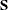matrix is computed as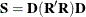where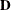is a diagonal matrix that depends on the VARDEF= option, the matrixis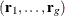, and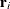is the vector of residuals for the ith equation with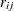replaced with zero whenis missing.

For MISSING=PAIRWISE, the calculation of the diagonal element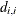of D is based on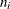, the number of nonmissing observations for the ith equation, instead of on n. Similarly, for VARDEF=WGT or WDF, the calculation is based on the sum of the weights for the nonmissing observations for the ith equation instead of on the sum of the weights for all observations. See the description of the VARDEF= option for the definition of D.

The degrees-of-freedom correction for a shared parameter is computed by using the average number of observations used in its estimation.

The MISSING=PAIRWISE option is not valid for the GMM and FIML estimation methods.

For the instrumental variables estimation methods (2SLS, 3SLS), when an instrument is missing for an observation, that observation is dropped for all equations, regardless of the MISSING= option.

#### PARMSDATA= Input Data Set

The option PARMSDATA= reads values for all parameters whose names match the names of variables in the PARMSDATA= data set. Values for any or all of the parameters in the model can be reset by using the PARMSDATA= option. The PARMSDATA= option goes in the PROC MODEL statement, and the data set is read before any FIT or SOLVE statements are executed.

In PROC MODEL, you have several options to specify starting values for the parameters to be estimated. When more than one option is specified, the options are implemented in the following order of precedence (from highest to lowest): the START= option, the PARMS statement initialization value, the ESTDATA= option, and the PARMSDATA= option. If no options are specified for the starting value, the default value of 0.0001 is used. Together, the OUTPARMS= and PARMSDATA= options enable you to change part of a model and recompile the new model program without the need to reestimate equations that were not changed.

Suppose you have a large model with parameters estimated and you now want to replace one equation, Y, with a new specification. Although the model program must be recompiled with the new equation, you don’t need to reestimate all the equations, just the one that changed.

Using the OUTPARMS= and PARMSDATA= options, you could do the following:

```   proc model model=oldmod outparms=temp; run;
proc model outmodel=newmod parmsdata=temp data=in;
...  include new model definition with changed y eq. here ...
fit y;
run;
```

The model file NEWMOD then contains the new model and its estimated parameters plus the old models with their original parameter values.

#### SDATA= Input Data Set

The SDATA= option allows a cross-equation covariance matrix to be input from a data set. The S matrix read from the SDATA= data set, specified in the FIT statement, is used to define the objective function for the OLS, N2SLS, SUR, and N3SLS estimation methods and is used as the initial S for the methods that iterate the S matrix.

Most often, the SDATA= data set has been created by the OUTS= or OUTSUSED= option in a previous FIT statement. The OUTS= and OUTSUSED= data sets from a FIT statement can be read back in by a FIT statement in the same PROC MODEL step.

You can create an input SDATA= data set by using the DATA step. PROC MODEL expects to find a character variable _NAME_ in the SDATA= data set as well as variables for the equations in the estimation or solution. For each observation with a _NAME_ value that matches the name of an equation, PROC MODEL fills the corresponding row of the S matrix with the values of the names of equations found in the data set. If a row or column is omitted from the data set, a 1 is placed on the diagonal for the row or column. Missing values are ignored, and since the S matrix is symmetric, you can include only a triangular part of the S matrix in the SDATA= data set with the omitted part indicated by missing values. If the SDATA= data set contains multiple observations with the same _NAME_, the last values supplied for the _NAME_ are used. The structure of the expected data set is further described in the section OUTS= Data Set.

Use the TYPE= option in the PROC MODEL or FIT statement to specify the type of estimation method used to produce the S matrix you want to input.

The following SAS statements are used to generate an S matrix from a GMM and a 3SLS estimation and to store that estimate in the data set GMMS:

```

proc model data=gmm2 ;
exogenous x1 x2;
parms a1 a2 b1 2.5 b2 c2 55 d1;
inst b1 b2 c2 x1 x2;
y1 = a1 * y2 + b1 * x1 * x1 + d1;
y2 = a2 * y1 + b2 * x2 * x2 + c2 / x2 + d1;

fit y1 y2 / 3sls gmm kernel=(qs,1,0.2)
outest=gmmest outs=gmms;
run;

proc print data=gmms;
run;
```

The data set GMMS is shown in Figure 19.67.

Figure 19.67: SDATA= Data Set

Obs _NAME_ _TYPE_ _NUSED_ y1 y2
1 y1 3SLS 50 27.1032 38.1599
2 y2 3SLS 50 38.1599 74.6253
3 y1 GMM 50 27.6248 32.2811
4 y2 GMM 50 32.2811 58.8387

#### VDATA= Input data set

The VDATA= option enables a variance matrix for GMM estimation to be input from a data set. When the VDATA= option is used in the PROC MODEL or FIT statement, the matrix that is input is used to define the objective function and is used as the initial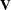for the methods that iterate thematrix.

Normally the VDATA= matrix is created from the OUTV= option in a previous FIT statement. Alternately an input VDATA= data set can be created by using the DATA step. Each row and column of thematrix is associated with an equation and an instrument. The position of each element in thematrix can then be indicated by an equation name and an instrument name for the row of the element and an equation name and an instrument name for the column. Each observation in the VDATA= data set is an element in thematrix. The row and column of the element are indicated by four variables (EQ_ROW, INST_ROW, EQ_COL, and INST_COL) that contain the equation name or instrument name. The variable name for an element is VALUE. Missing values are set to 0. Because the variance matrix is symmetric, only a triangular part of the matrix needs to be input.

The following SAS statements are used to generate a V matrix estimation from GMM and to store that estimate in the data set GMMV:

```

proc model data=gmm2;
exogenous x1 x2;
parms a1 a2 b2 b1 2.5 c2 55 d1;
inst b1 b2 c2 x1 x2;
y1 = a1 * y2 + b1 * x1 * x1 + d1;
y2 = a2 * y1 + b2 * x2 * x2 + c2 / x2 + d1;

fit y1 y2 / gmm outv=gmmv;
run;
```
```proc print data=gmmv(obs=15);
run;
```

The data set GMM2 was generated by the example in the preceding ESTDATA= section. The V matrix stored in GMMV is selected for use in an additional GMM estimation by the following FIT statement:

```   fit y1 y2 / gmm vdata=gmmv;
run;
```

A partial listing of the GMMV data set is shown in Figure 19.68. There are a total of 78 observations in this data set. The V matrix is 12 by 12 for this example.

Figure 19.68: The First 15 Observations in the VDATA= Data Set

Obs _TYPE_ EQ_ROW EQ_COL INST_ROW INST_COL VALUE
1 GMM y1 y1 1 1 1555.78
2 GMM y1 y1 x1 1 8565.80
3 GMM y1 y1 x1 x1 49932.47
4 GMM y1 y1 x2 1 8244.34
5 GMM y1 y1 x2 x1 51324.21
6 GMM y1 y1 x2 x2 159913.24
7 GMM y1 y1 @PRED.y1/@b1 1 49933.61
8 GMM y1 y1 @PRED.y1/@b1 x1 301270.02
9 GMM y1 y1 @PRED.y1/@b1 x2 317277.10
10 GMM y1 y1 @PRED.y1/@b1 @PRED.y1/@b1 1860095.90
11 GMM y1 y1 @PRED.y2/@b2 1 163855.31
12 GMM y1 y1 @PRED.y2/@b2 x1 900622.60
13 GMM y1 y1 @PRED.y2/@b2 x2 1285421.56
14 GMM y1 y1 @PRED.y2/@b2 @PRED.y1/@b1 5173744.58
15 GMM y1 y1 @PRED.y2/@b2 @PRED.y2/@b2 30307640.16